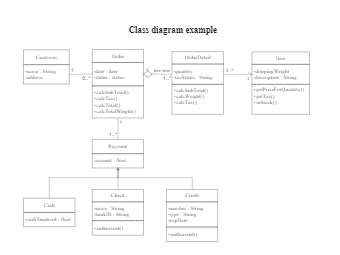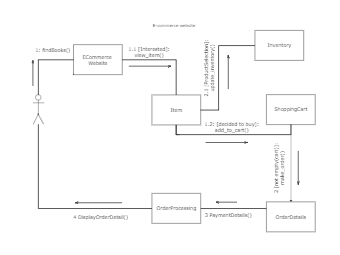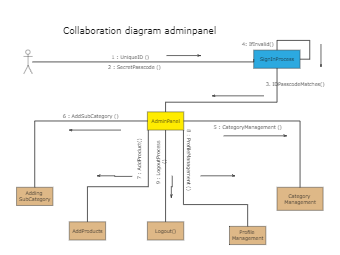## Order System UML Class Diagram

852
0
8
publish time: 2020-09-11lizzie

Here is a UML class diagram that shows how an order system works. The class in UML diagrams describes a concept that expresses the state (attributes) and behavior (operations). The class is a blueprint for an object, and an object is an instance of a class. You can create a UML class diagram like this class in EdrawMax as it provides built-in and industry-standard symbols.

See More Related TemplatesThis is an example of a UML diagram about adding events to the calendar.
UML Diagram - Add event to calendar
24
1
0easy diagramsThis is an example of a uml class diagram.
Class diagram example
209
6
4easy diagramsThis is an example of a UML collaboration diagram.
ecommerce website - UML diagram
26
2
0easy diagramseasy diagrams